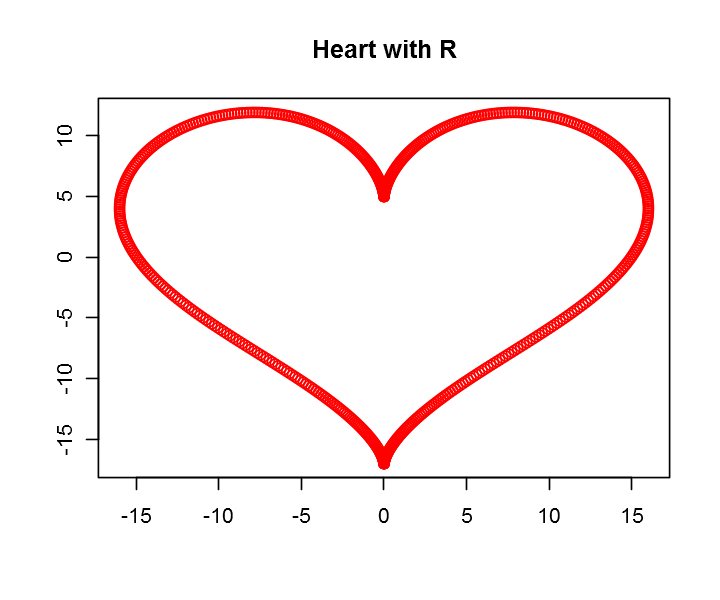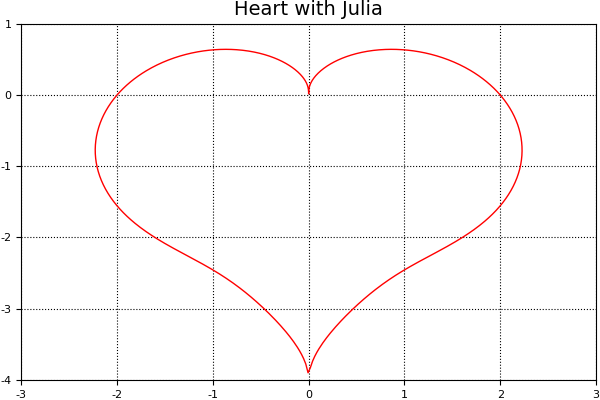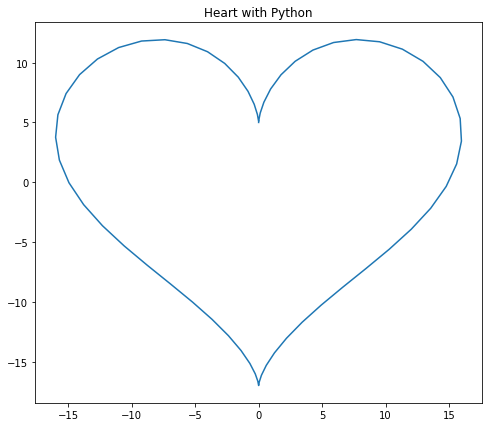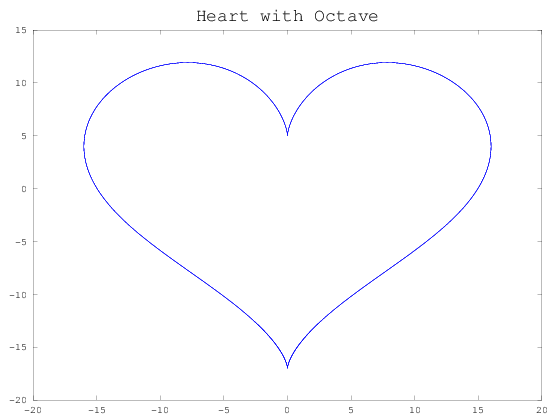# Fisseha Berhane, PhD

#### Data Scientist443-970-2353[email protected] CV Resume### Using Python, R, Julia and Octave with Jupyter Notebook¶

Jupyter Notebook is an ideal tool for data cleaning and transformation, numerical simulation, statistical modeling, machine learning, etc. It helps to create and share documents that contain narration, code and code output.

Jupyter Notebook has support for over 40 programming languages, including those popular in Data Science such as Python, R, Julia, and Scala. Since it supports Spark, it can be used for big data analytics.

You can create interactive visualizations, presentations and other documents such as your research thesis in a Jupyter Notebook with one or a combination of the programing languages it supports. Jupyter Notebook helps to easily share your work with the world by exporting all your work as html and putting it on your blog, or Github for example. You can also export it as pdf.

The video tutorial below shows how to intall Jupyter from Anaconda and how to add R, Julia and Octave kernels. If you want to export your work in Jupyter Notebbok as pdf document, you may need to install MiKTeX. The codes I used to create plots are shown below the YouTube video.

Let's plot heart shapes using R, Julia, Octave and Python on the same Jupyter Notebook session.

### R¶

In :
options(repr.plot.width = 6)
options(repr.plot.height = 5)

t = seq(0, 2*pi, by = 0.01)
x = 16 * sin(t)^3
y = 13 * cos(t) - 5*cos(2*t) - 2*cos(3*t) - cos(4*t)
plot(x, y, main = "Heart with R", xlab = "", ylab = "", col = "red")### Julia¶

In :
using Plots
r(θ)=sin(θ).*√(abs(cos(θ)))./(sin(θ)+7/5)-2sin(θ)+2

for i in -6:6
θ=π+i*π/6;
y=r(θ);
end

θ=linspace(0,2*π,1000)
x=r(θ).*cos(θ)
y=r(θ).*sin(θ)

pyplot()
plot(x,y, linecolor=:red, leg=false)
ax = PyPlot.gca()
ax[:set_xlim]((-3,3));
ax[:set_ylim]((-4,1));
plot(x,y, linecolor=:red, leg=false, xlims = (-3,3), ylims=(-4,1), title = "Heart with Julia")

Out:### Python¶

In :
import matplotlib.pyplot as plt
import numpy as np
plt.figure(figsize = [8, 7])
t = np.arange(0,2*np.pi, 0.1)
x = 16*np.sin(t)**3
y = 13*np.cos(t)-5*np.cos(2*t)-2*np.cos(3*t)-np.cos(4*t)
plt.plot(x,y)
plt.title("Heart with Python")

Out:
<matplotlib.text.Text at 0x74b7a20>
In :
plt.show()### Octave¶

In :
t=-10:0.01:10;
x=16*(power(sin(t),3));
y=(13*cos(t))-(5*cos(2*t))-(2*cos(3*t))-(cos(4*t));
plot(x,y)
title("Heart with Octave", 'Color','k','fontsize',18)